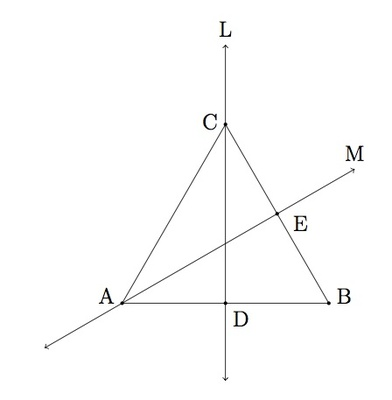# Reflections and Equilateral Triangles II

Alignments to Content Standards: G-CO.B

Suppose $ABC\,$ is an equilateral triangle. Let $D$ be the midpoint of side $\overline{AB}$ and $E$ the midpoint of side $\overline{BC}$ as pictured below:The line through $C$ and $D$ is denoted $L$ and the line through $E$ and $A$ is denoted $M$. Let $r_L$ denote the reflection of the plane about the line $L$ and $r_M$ reflection of the plane about the line $M$.

1. Where do the reflections $r_L$ and $r_M$ map the vertices $A,B,$ and $C$ of triangle $ABC$? Explain.
2. Where do the vertices $A,B,$ and $C$ end up if the plane is first reflected about line $M$ and then about $L$?
3. Where do the vertices $A,B,$ and $C$ end up if the plane is first reflected about line $L$ and then about $M$? Is the answer the same as (b) or different?

## IM Commentary

This task examines some of the properties of reflections of the plane which preserve an equilateral triangle: these were introduced in ''Reflections and Isosceles Triangles'' and ''Reflection and Equilateral Triangles I''. The task gives students a chance to see the impact of these reflections on an explicit object and to see that the reflections do not always commute, that is $r_L(r_M(P))$ is not the same as $r_M(r_L(P))$ unless $P$ is the center of the triangle: the first of these rotates 120 counterclockwise while the other rotates 120 degrees clockwise. The solution to this task uses the fact, established in ''Reflections and triangles I, II,'' that the lines $L$ and $M$ are the perpendicular bisectors of segments $\overline{AB}$ and $\overline{BC}$ respectively.

In order to help visualize how the different reflections of the plane influence the vertices of a triangle, students may wish to use manipulatives: this can either take the form of paper triangle cutouts with labelled vertices which can either be folded along the line of reflection or actually reflected. Both approaches are important: if using the latter, however, students need to be careful to record where the vertices of the triangle were prior to the reflection. This will also be important as they continue to reflect because the three lines of reflection of this problem are all determined by the original triangle rather than its reflected images.

The problem can be naturally extended. Suppose $F$ is the midpoint of side $\overline{AC}$ and $K$ the line joining $B$ and $F$. Let $r_K$ denote reflection about $F$. Then the students can be asked, for example, to write $r_F$ in terms of $r_L$ and $r_M$. Geometrically, if the triangle is rotated counterclockwise by $120$ degrees about its center then line $K$ gets mapped to line $L$. So rotating $120$ degrees counterclockwise and then reflecting about $L$ will have the same effect as reflecting about $K$. If $r_K$ denotes reflection about $K$ this means that $$r_K(x) = r_L(r_M(r_L(x)))$$ for every $x$ in the triangle $ABC$.

In fact, all symmetries of the triangle $ABC$, that is all rigid transformations of the plane which preserve triangle $ABC$, can be expressed in terms of the reflections $r_L$ and $r_M$ as was done in the previous paragraph for $r_K$. This task is primarily intended for instructional purposes, providing a context for students to explore how reflections of the plane behave under composition. If used for assessment, it is vital that students have already mastered the material from ''Reflections and Isosceles Triangles'' and ''Reflection and Equilateral Triangles I.''

## Solution

1. The line $L$ passes through vertex $C$ of the triangle. Since points on $L$ are all fixed when reflecting about $L$, we have $r_L(C) = C$. Since $L$ is the perpendicular bisector of segment $\overline{AB}$, reflection about $L$ will map $B$ to $C$ and $C$ to $B$ so $r_L(B) = A$ and $r_L(A) = B$.
2. In order to see what happens when two successive reflections are performed, we first check the impact of reflection about $M$ on the vertices. Since $A$ lies on $M$ we know that $r_M(A) = A$. Since $M$ is the perpendicular bisector of $\overline{BC}$ we have $r_M(B) = C$ and $r_M(C) = B$. We have calculated in part (a) the impact of $r_L$ on the three vertices so we can calculate: \begin{eqnarray} r_M(r_L(A)) &=& r_M(B) = C \\ r_M(r_L(B)) &=& r_M(A) = A\\ r_M(r_L(C)) &=& r_M(C) = B. \end{eqnarray} Sending $A$ to $C$, $C$ to $B$, and $B$ to $A$ can also be accomplished by rotating the triangle by $120$ clockwise about its center (the point where $L$ and $M$ meet). So the net effect of reflecting about $M$ and then reflecting about $L$ is a rotation.

3. Reversing the order of the two reflections from part (b) we find \begin{eqnarray} r_L(r_M(A)) &=& r_L(A) = B \\ r_L(r_M(B)) &=& r_L(C) = C\\ r_L(r_M(C)) &=& r_L(B) = A. \end{eqnarray}

The calculations above show that performing reflection about $M$ and then reflection about $L$ does not have the same impact on the triangle $ABC$ as performing reflection about $L$ and then reflection about $M$: the order in which the reflections are performed influences the outcome.

Note that performing $r_L$ and then $r_M$ undoes the transformation obtained by performing $r_M$ and then $r_L$. This is because performing a reflection about the same line twice moves all points back to their original position: \begin{eqnarray} r_L(r_M(r_M(r_L(x)))) &=& r_L(r_L(x)) = x \\ r_M(r_L(r_L(r_M(x)))) &=& r_M(r_M(x)) = x \end{eqnarray} for any $x$ in the plane.